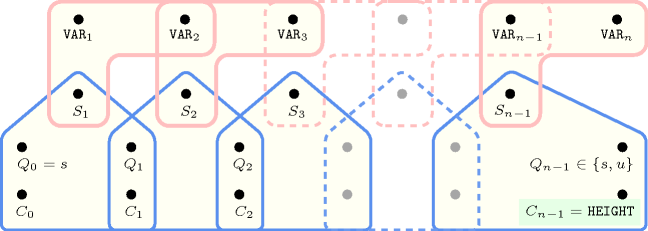## 5.175. highest_peak

Origin

Derived from $\mathrm{𝚙𝚎𝚊𝚔}$.

Constraint

$\mathrm{𝚑𝚒𝚐𝚑𝚎𝚜𝚝}_\mathrm{𝚙𝚎𝚊𝚔}\left(\mathrm{𝙷𝙴𝙸𝙶𝙷𝚃},\mathrm{𝚅𝙰𝚁𝙸𝙰𝙱𝙻𝙴𝚂}\right)$

Arguments
 $\mathrm{𝙷𝙴𝙸𝙶𝙷𝚃}$ $\mathrm{𝚍𝚟𝚊𝚛}$ $\mathrm{𝚅𝙰𝚁𝙸𝙰𝙱𝙻𝙴𝚂}$ $\mathrm{𝚌𝚘𝚕𝚕𝚎𝚌𝚝𝚒𝚘𝚗}\left(\mathrm{𝚟𝚊𝚛}-\mathrm{𝚍𝚟𝚊𝚛}\right)$
Restriction
$\mathrm{𝚛𝚎𝚚𝚞𝚒𝚛𝚎𝚍}$$\left(\mathrm{𝚅𝙰𝚁𝙸𝙰𝙱𝙻𝙴𝚂},\mathrm{𝚟𝚊𝚛}\right)$
Purpose

A variable ${V}_{k}$ $\left(1 of the sequence of variables $\mathrm{𝚅𝙰𝚁𝙸𝙰𝙱𝙻𝙴𝚂}={V}_{1},\cdots ,{V}_{m}$ is a peak if and only if there exists an $i$ $\left(1 such that ${V}_{i-1}<{V}_{i}$ and ${V}_{i}={V}_{i+1}=\cdots ={V}_{k}$ and ${V}_{k}>{V}_{k+1}$. $\mathrm{𝙷𝙴𝙸𝙶𝙷𝚃}$ is the maximum value of the peak variables. If no such variable exists $\mathrm{𝙷𝙴𝙸𝙶𝙷𝚃}$ is equal to $\mathrm{𝙼𝙸𝙽𝙸𝙽𝚃}$.

Example
 $\left(8,〈1,1,4,8,6,2,7,1〉\right)$ $\left(1,〈0,1,1,0,0,1,0,1〉\right)$

The first $\mathrm{𝚑𝚒𝚐𝚑𝚎𝚜𝚝}_\mathrm{𝚙𝚎𝚊𝚔}$ constraint holds since 8 is the maximum peak of the sequence $11486271$.

##### Figure 5.175.1. Illustration of the first constraint of the Example slot: a sequence of eight variables ${V}_{1}$, ${V}_{2}$, ${V}_{3}$, ${V}_{4}$, ${V}_{5}$, ${V}_{6}$, ${V}_{7}$, ${V}_{8}$ respectively fixed to values 1, 1, 4, 8, 6, 2, 7, 1 and its corresponding highest peak 8Typical
 $|\mathrm{𝚅𝙰𝚁𝙸𝙰𝙱𝙻𝙴𝚂}|>2$ $\mathrm{𝚛𝚊𝚗𝚐𝚎}$$\left(\mathrm{𝚅𝙰𝚁𝙸𝙰𝙱𝙻𝙴𝚂}.\mathrm{𝚟𝚊𝚛}\right)>2$ $\mathrm{𝚙𝚎𝚊𝚔}$$\left(\mathrm{𝚅𝙰𝚁𝙸𝙰𝙱𝙻𝙴𝚂}.\mathrm{𝚟𝚊𝚛}\right)>0$
Symmetry

Items of $\mathrm{𝚅𝙰𝚁𝙸𝙰𝙱𝙻𝙴𝚂}$ can be reversed.

Arg. properties

Functional dependency: $\mathrm{𝙷𝙴𝙸𝙶𝙷𝚃}$ determined by $\mathrm{𝚅𝙰𝚁𝙸𝙰𝙱𝙻𝙴𝚂}$.

Counting
 Length ($n$) 2 3 4 5 6 7 8 Solutions 9 64 625 7776 117649 2097152 43046721

Number of solutions for $\mathrm{𝚑𝚒𝚐𝚑𝚎𝚜𝚝}_\mathrm{𝚙𝚎𝚊𝚔}$: domains $0..n$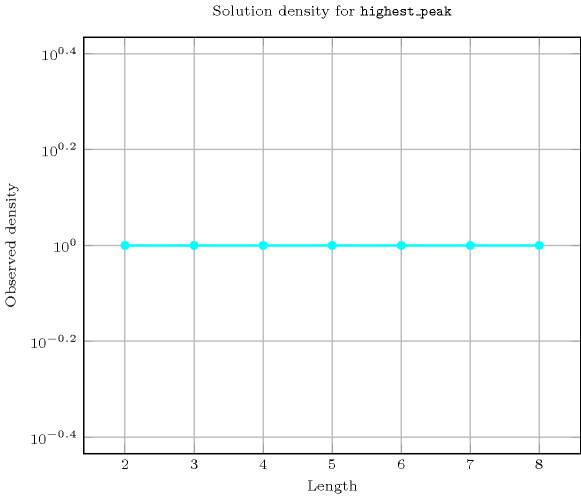Length ($n$)2345678
Total9646257776117649209715243046721
 Parameter value

-100000095029517921108869498439791
1-11192697503635443
2-444380300022632166208
3-999900758761389484020
4--1761712156801385441195056
5---2900291252832502693425
6----504725405765665896
7-----97622711233250
8------21133632

Solution count for $\mathrm{𝚑𝚒𝚐𝚑𝚎𝚜𝚝}_\mathrm{𝚙𝚎𝚊𝚔}$: domains $0..n$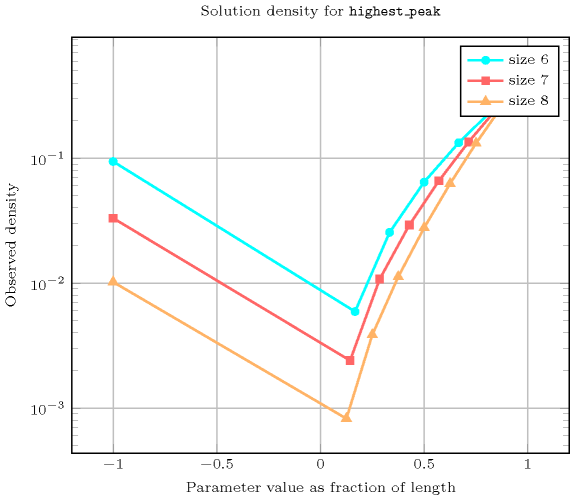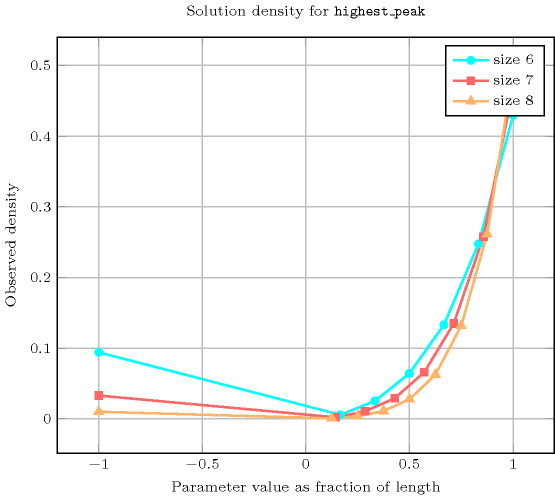Figure 5.175.2 depicts the automaton associated with the $\mathrm{𝚑𝚒𝚐𝚑𝚎𝚜𝚝}_\mathrm{𝚙𝚎𝚊𝚔}$ constraint. To each pair of consecutive variables $\left({\mathrm{𝚅𝙰𝚁}}_{i},{\mathrm{𝚅𝙰𝚁}}_{i+1}\right)$ of the collection $\mathrm{𝚅𝙰𝚁𝙸𝙰𝙱𝙻𝙴𝚂}$ corresponds a signature variable ${S}_{i}$. The following signature constraint links ${\mathrm{𝚅𝙰𝚁}}_{i}$, ${\mathrm{𝚅𝙰𝚁}}_{i+1}$ and ${S}_{i}$:
${\mathrm{𝚅𝙰𝚁}}_{i}<{\mathrm{𝚅𝙰𝚁}}_{i+1}⇔{S}_{i}=0\wedge {\mathrm{𝚅𝙰𝚁}}_{i}={\mathrm{𝚅𝙰𝚁}}_{i+1}⇔{S}_{i}=1\wedge {\mathrm{𝚅𝙰𝚁}}_{i}>{\mathrm{𝚅𝙰𝚁}}_{i+1}⇔{S}_{i}=2$.
##### Figure 5.175.2. Automaton of the $\mathrm{𝚑𝚒𝚐𝚑𝚎𝚜𝚝}_\mathrm{𝚙𝚎𝚊𝚔}$ constraint and its glue matrix (state $s$ means that we are in decreasing or stationary mode, state $u$ means that we are in increasing mode, a new peak is detected each time we switch from increasing to decreasing mode and the counter $C$ is updated accordingly); $\text{minint}$ is the smallest integer that can be represented on a machine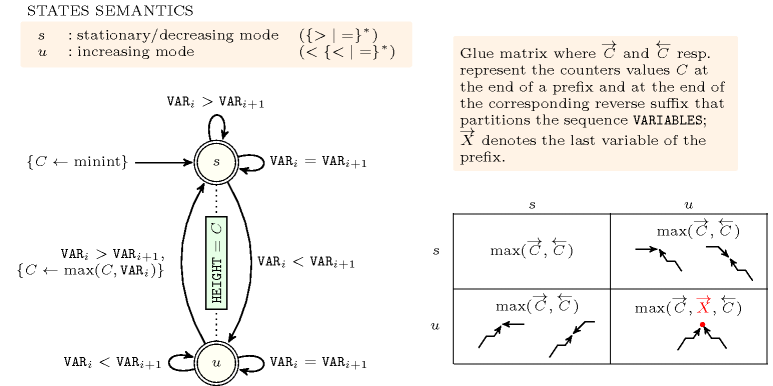##### Figure 5.175.3. Hypergraph of the reformulation corresponding to the automaton of the $\mathrm{𝚑𝚒𝚐𝚑𝚎𝚜𝚝}_\mathrm{𝚙𝚎𝚊𝚔}$ constraint (${C}_{0}$ is set to $\text{minint}$ the largest integer that can be represented on a machine)